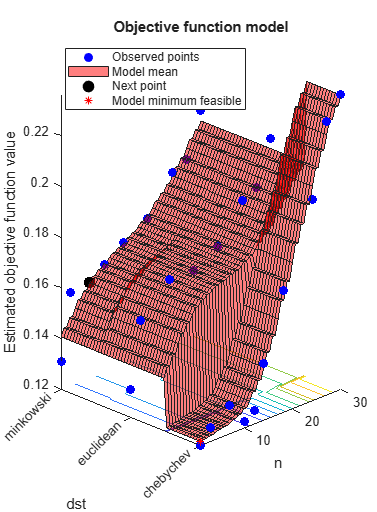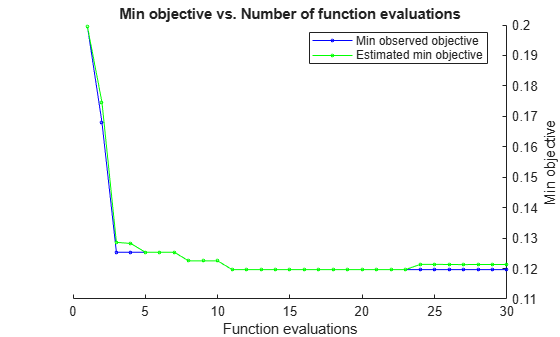Documentation

predictObjective

Predict objective function at a set of points

Description

example

objective = predictObjective(results,XTable) returns the estimated objective function value at the points in XTable.

example

[objective,sigma] = predictObjective(results,XTable) also returns estimated standard deviations.

Examples

collapse all

This example shows how to estimate the cross-validation loss of an optimized classifier.

Optimize a KNN classifier for the ionosphere data, meaning find parameters that minimize the cross-validation loss. Minimize over nearest-neighborhood sizes from 1 to 30, and over the distance functions 'chebychev', 'euclidean', and 'minkowski'.

For reproducibility, set the random seed, and set the AcquisitionFunctionName option to 'expected-improvement-plus'.

rng default
num = optimizableVariable('n',[1,30],'Type','integer');
dst = optimizableVariable('dst',{'chebychev','euclidean','minkowski'},'Type','categorical');
c = cvpartition(351,'Kfold',5);
fun = @(x)kfoldLoss(fitcknn(X,Y,'CVPartition',c,'NumNeighbors',x.n,...
'Distance',char(x.dst),'NSMethod','exhaustive'));
results = bayesopt(fun,[num,dst],'Verbose',0,...
'AcquisitionFunctionName','expected-improvement-plus');Create a table of points to estimate.

b = categorical({'chebychev','euclidean','minkowski'});
n = [1;1;1;4;2;2];
dst = [b(1);b(2);b(3);b(1);b(1);b(3)];
XTable = table(n,dst);

Estimate the objective and standard deviation of the objective at these points.

[objective,sigma] = predictObjective(results,XTable);
[XTable,table(objective,sigma)]
ans=6×4 table
n       dst       objective      sigma
_    _________    _________    _________

1    chebychev     0.12132     0.0068029
1    euclidean     0.14052     0.0079128
1    minkowski     0.14057     0.0079117
4    chebychev      0.1227     0.0068805
2    chebychev     0.12176     0.0066739
2    minkowski      0.1437     0.0075448

Input Arguments

collapse all

Bayesian optimization results, specified as a BayesianOptimization object.

Prediction points, specified as a table with D columns, where D is the number of variables in the problem. The function performs its predictions on these points.

Data Types: table

Output Arguments

collapse all

Objective estimates, returned as an N-by-1 vector, where N is the number of rows of XTable. The estimates are the mean values of the posterior distribution of the Gaussian process model of the objective function.

Standard deviations of objective function, returned as an N-by-1 vector, where N is the number of rows of XTable. The standard deviations are those of the posterior distribution of the Gaussian process model of the objective function.